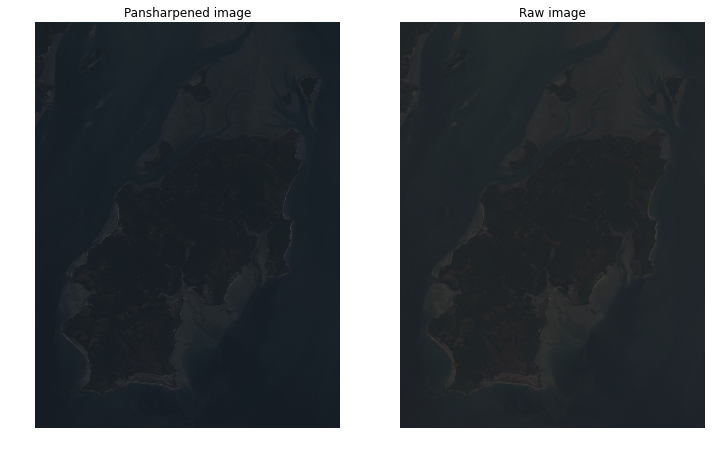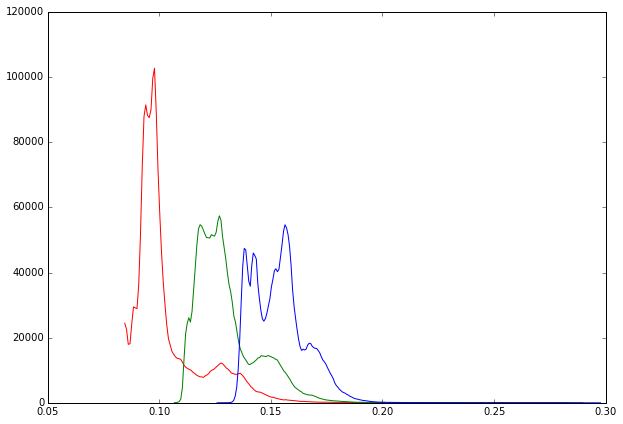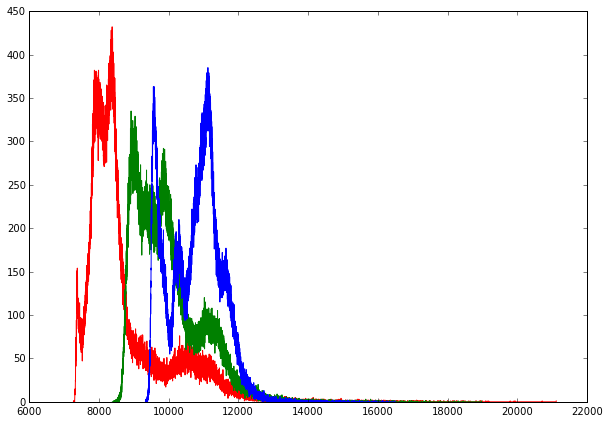# Panchromatic Sharpening¶

This notebook is part of the collection accompanying the talk "Analyzing Satellite Images With Python Scientific Stack" by Milos Miljkovic given at PyData NYC 2014. The content is BSD licensed.

Panchromatic sharpening is a radiometric transformation which uses a higher resolution panchromatic band to fuse it with lower resolution red-green-blue bands. In other words, spatial information in the high-resolution grayscale Band 8 and color information in the RGB color Bands 4, 3, and 2 is merged to create a high-resolution color image.

There are a few algorithms for sharpening: Brovey, IHS, PCA, Gram-Schmidt, simple mean, and weighted algorithms used on per satellite data sets.

## Brovey Algorithm¶

The Brovey algorithm is a transform which has the properties of conserving image resolution and slightly skewing the color balance by increasing the low and high ends in image histograms.

In :
# To install watermark extension execute:
# %install_ext https://raw.githubusercontent.com/HyperionAnalytics/watermark/master/watermark.py

In :
%watermark -v -m -p numpy,scikit-image,matplotlib

CPython 3.4.2
IPython 2.3.1

numpy 1.9.1
scikit-image 0.10.1
matplotlib 1.4.2

compiler   : GCC 4.2.1 (Apple Inc. build 5577)
system     : OS X 10.10.1
release    : 14.0.0
machine    : x86_64
processor  : Intel(R) Core(TM) i7-3740QM CPU @ 2.70GHz
CPU cores  : 8
interpreter: 64bit

In :
cd /Users/kronos/gis/l8/guinea_bissau/

/Users/kronos/gis/l8/guinea_bissau

In :
import numpy as np
from skimage import io, transform, exposure
from matplotlib import pyplot as plt

In :
%matplotlib inline

In :
def read_band(n):
"""
Input:
n - integer in the range 1-11
Output:
img - 2D array of uint16 type
"""
if n in range(1, 12):
tif_list = !ls *.TIF
band_name = 'B' + str(n) + '.TIF'
img_idx = [idx for idx, band_string in enumerate(tif_list) if band_name in band_string]
return img
else:
print('Band number has to be in the range 1-11!')

In :
b4 = read_band(4)

/Users/kronos/anaconda3/lib/python3.4/site-packages/PIL/Image.py:2192: DecompressionBombWarning: Image size (220222881 pixels) exceeds limit of 89478485 pixels, could be decompression bomb DOS attack.
DecompressionBombWarning)

In :
img432_roi = np.dstack((b4, b3, b2))[4400:5200, 3500:4100, :]


### Scale all the bands to 0 - 1 range.¶

We will use only a portion of the sample image to ease RAM requirements and make transform more apparent.

In :
b4 = b4/b4.max()
b3 = b3/b3.max()
b2 = b2/b2.max()
b8 = b8/b8.max()

In :
b4 = b4[4400:5200, 3500:4100]
b3 = b3[4400:5200, 3500:4100]
b2 = b2[4400:5200, 3500:4100]
b8 = b8[8800:10400, 7000:8200]


### Rescale RGB color image to match the resolution of the pan band.¶

In :
img432 = np.dstack((b4, b3, b2))
img432_2x = transform.rescale(img432, 2)


### Divide each of the up-sampled RGB bands by their sum and multiply by pan band to produce three high-resolution RGB channels.¶

In :
m = np.sum(img432_2x, axis=2)
ps4 = b8*img432_2x[:, :, 0]/m
ps3 = b8*img432_2x[:, :, 1]/m
ps2 = b8*img432_2x[:, :, 2]/m
img432_ps = np.dstack((ps4, ps3, ps2))

In :
fig = plt.figure(figsize=(12, 9))
fig.set_facecolor('white')

ax1.imshow(img432_ps)
plt.title('Pansharpened image')
plt.axis('off')

ax2.imshow(img432_roi/65535)
plt.title('Raw image')
plt.axis('off')

plt.show()In :
fig = plt.figure(figsize=(10, 7))
fig.set_facecolor('white')

for color, channel in zip('rgb', np.rollaxis(img432_ps, axis=-1)):
counts, centers = exposure.histogram(channel)
plt.plot(centers[1::], counts[1::], color=color)

plt.show()In :
fig = plt.figure(figsize=(10, 7))
fig.set_facecolor('white')

for color, channel in zip('rgb', np.rollaxis(img432_roi, axis=-1)):
counts, centers = exposure.histogram(channel)
plt.plot(centers[1::], counts[1::], color=color)

plt.show()In :
img1 = np.empty(img432_ps.shape, dtype='float64')
lims = [(0.088,0.17), (0.108, 0.19), (0.130,0.20)]
for lim, channel in zip(lims, range(3)):
img1[:, :, channel] = exposure.rescale_intensity(img432_ps[:, :, channel], lim)

In :
img2 = np.empty(img432_roi.shape, dtype='float64')
lims = [(7100,14500), (8200, 14000), (9200,13500)]
for lim, channel in zip(lims, range(3)):
img2[:, :, channel] = exposure.rescale_intensity(img432_roi[:, :, channel], lim)

In :
fig = plt.figure(figsize=(12, 9))
fig.set_facecolor('white')